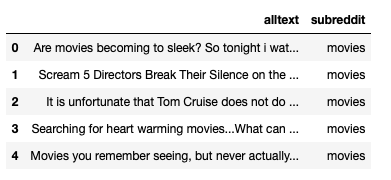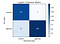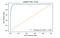# Classifying Text Content with TF-IDF

In Natural Language Processing (NLP), word vectorization offers several options for assigning numerical values to text data. It is this process that allows us to build models that can reliably identify characteristics from a given document. We will explore how Term Frequency-Inverse Document Frequency (TF-IDF) vectorization can be applied to distinguish patterns in a document and help us classify where text may have originated from given its content.

Background for Experiment:
We will be using TF-IDF to help us classify content from Reddit posts to see if a model can identify which subreddit a post came from. For our purposes here, we have selected the Movies and Gaming subreddits. Both of these subreddits are distinct enough, but also overlap in that they both refer to entertainment topics. This can be sufficiently challenging for a classification model and an opportunity to see what TF-IDF can do for us.

Reddit Text Data:
We used the PRAW API for retrieving the text data from the respective subreddits. In advance of vectorization we created two DataFrames for the Movies (dfm), and Gaming (dfg) subreddits. Below is an example of our text data and target to be later classified for the “movies” subreddit:‘alltext’ was engineered to combine both the title and body text of the post.

TF-IDF: Background & Application
Vectorizing the words demands a few prior considerations. Do we leave in or remove stop words? (and, the, to, on etc.), What can we learn from the most common N-gram ranges in our data? N-grams are groupings of N amount of words that themselves can reveal interesting patterns about text data. TF-IDF assigns a score to particular words or N-grams based on whether it occurs more or less frequently in a document. We can think of a TF-IDF score as a measure of the relative importance of a word vector when comparing between different document types. Another discription of this is “document similarity” with similarity here being measured by how common or “important” certain words are in one document vs. another.

Vectorization & Modeling:
Can TF-IDF help us make a robust model for classifying between different Subreddits? Lets find out. We’ll be using Scikit-learn’s Pipeline and GridSearchCV to fully utilize the TF-IDF transformer. We will be using a basic Logistic Regression model to classify the documents:

`# import librariesimport matplotlib.pyplot as pltfrom sklearn.feature_extraction.text import TfidfVectorizerfrom sklearn.model_selection import GridSearchCV from sklearn.linear_model import LogisticRegressionfrom sklearn.pipeline import Pipeline# Pipeline for TF-IDF with Logistic Regression modelpipe_tvec = Pipeline([    ('tvec', TfidfVectorizer()),    ('lr', LogisticRegression())])# Parameters for TF-IDF vectorization for GridSearchpipe_tvec_params = {    'tvec__max_features': [2_000, 3_000, 4_000, 5_000],    'tvec__stop_words': [None, 'english'],    'tvec__ngram_range': [(1,1), (1,2)]}# Initialize and fit GridSearchgs_tvec = GridSearchCV(pipe_tvec,param_grid = pipe_tvec_paramscv=5)gs_tvec.fit(X_train,y_train)`

After fitting our model with GridSearch, we can create and evaluate the classification metrics. We’ll take a look at the Confusion martix and ROC Curve.

`from sklearn.metrics import confusion_matrix, plot_confusion_matrix, plot_roc_curve# Generate predictions and create confusion matrixpreds_tvec = gs_tvec.predict(X_test)tn, fp, fn, tp = confusion_matrix(y_test, preds_tvec).ravel()# Display confusion matrixplot_confusion_matrix(gs_cvec,X_test,y_test,cmap='Blues',values_format='d',display_labels=['movies','gaming'])plt.title('Logistic Confusion Matrix');`Our model did very well at distinguishing the two classes with minimal counts for both false positives and false negatives

We have encouraging results from the Confusion Matrix from TF-IDF being paired with Logistic Regression. Let’s see if this is also validated by the ROC Curve:

`plot_roc_curve(gs_cvec,X_test,y_test)plt.plot([0,1],[0,1],        label='baseline',linestyle='--')plt.title('Logistic ROC Curve')plt.legend;`This is a great performance by the model, with a possible max AUC score of 1

For good measure, let’s also take a look at the other ratios of true/false positive/negative rates:

`print('precision: ',tp / (tp + fp))print('sensitivity: ',tp / (tp + fn))print('specificity: ',tn / (tn + fp))print('accuracy: ', (tn + tp) / (tn + fp + fn + tp))`

What did we find was the best hyperpeter combination for TF-IDF?

`gs_tvec.best_params_`

Summary:
We can definitely appreciate the nuances offered by TF-IDF. It intuitevely makes sense that different words would have more “importance” in different documents and our transformation of that importance into scores has delivered a resonably good classifier. We can proceed with different types of classification models following this to see if our results could be even better.